# A second (more elegant) proof of the Quotient Rule

The quotient rule proof can be quite tedious if we are only allowed to use the definition of the derivative. In this post, this presents an alternative way of proving the quotient rule if we are allowed to use the product rule.

The Quotient Rule: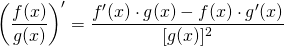Let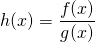be the quotient of differentiable functions. Then, we can rearrange so that we have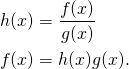Assuming that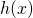is differentiable, we can use the product rule on, which yieldsThen we isolate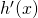on one side of the equation.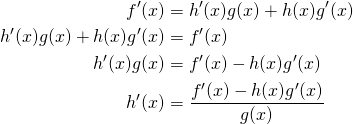Now, we substitute.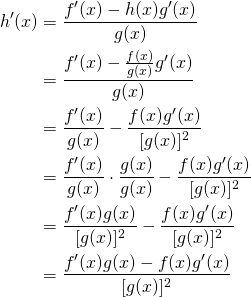We have arrived at the desired result, and this completes our proof.

Tags: , ,